##### Common Core Math For Parents For Dummies with Videos Online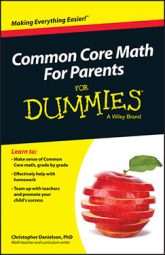Math students add and multiply fractions in Common Core fifth grade. These skills require using and extending their knowledge of these operations from their earlier work with whole numbers.

When adding two numbers, fifth graders need to express the same units. Three miles plus four gallons doesn't give you seven of anything because the units are different, which is true with adding fractions, too. When students get to fifth grade, they have a lot of experience with unit fractions. A unit fraction is a fraction with a 1 in the numerator, such as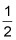or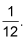The unit in unit fraction refers to the 1 in the numerator. But a unit fraction is also a unit just like other units of measurement. A mile is a unit, a gallon is a unit, and a fourth is a unit. It's helpful for students to think about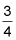as three fourths, just like they think of three miles and three gallons. This can remind them that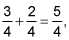and — more generally — that adding fractions requires common denominators because you need to add same-sized units.

The hard part about multiplying fractions isn't the algorithm. It's an easy algorithm: Just multiply the numerators and multiply the denominators.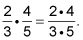Nor is the idea behind fraction multiplication particularly difficult to understand. When you multiply two fractions, you're finding parts of parts. When you multiply a fraction by a whole number, you're finding part of that whole number — half of 12,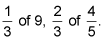Students draw pictures to solve problems like in this figure that involve parts of parts.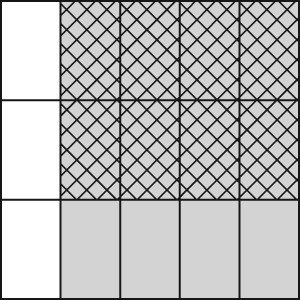Finding two-thirds of four-fifths.

You can see the doubly shaded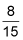in the upper-right part of the diagram.

This product refers to a fraction of the original whole — the square in the figure.